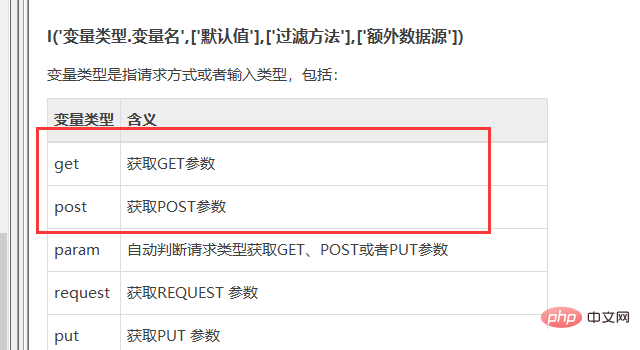# thinkphp中常用的提交表单的方法IS_POST 如果请求是post，则IS_POST的值是true，否则是false

IS_GET

IS_AJAX 如果请求是ajax，则IS_AJAX的值是true，否则是false

IS_CGI

IS_PUT```<?php
if(IS_POST){
//处理表单提交
\$post = I('post.');
//添加数据
//实例化模型
\$model = M('Dept');
//判断返回值
if(\$result){
//添加成功
\$this -> success('添加成功',U('showList'),5);
}else{
\$this -> error('添加失败');
}
}else{
//实例化模型
\$model = M('Dept');
//查询操作
\$data = \$model -> where('pid = 0') -> select();
//变量分配
\$this -> assign('data',\$data);
//展示模板
\$this -> display();
}
}```